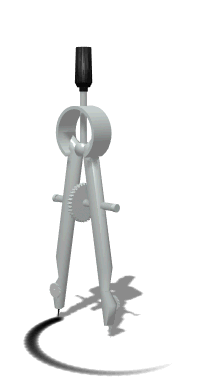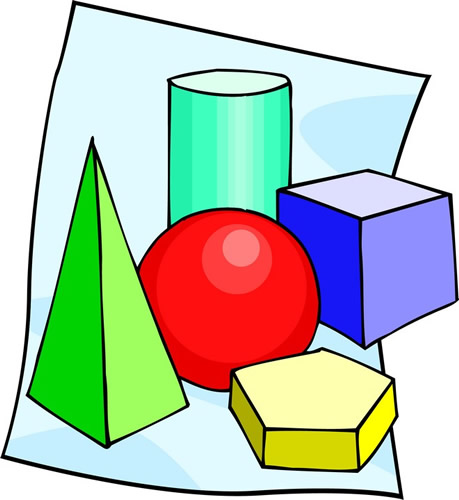•Fourth Grade Bridges Math ProgramWe will be working with our new Grade 4 program of Bridges in Mathematics.  It contains many exciting and challenging features for everyone! In grade 4 math, your child will: understand factors and multiples, e.g., 1,2,4,5,10, and 20 are all factors of 20, and 20 is a multiple of each of those numbers understand that a prime number has only 2 factors—1 and itself, while a composite number has more than 2 factors multiply multi-digit numbers using strategies based on place value and properties of operations divide 2-digit numbers by 1-digit numbers using strategies based on place value and the relationship between multiplication and division read, write, and compare multi-digit numbers and round multi-digit numbers to any place add and subtract multi-digit numbers accurately and efficiently recognize and generate equivalent fractions compare two fractions with different numerators and denominators add and subtract fractions and mixed numbers with like denominators multiply a fraction by a whole number write fractions with denominators of 10 or 100 in decimal notation compare decimal numbers with digits to the hundredths place know the relative sizes of measurement units within one system of units, including metric length, metric mass, customary weight, metric volume, and time use formulas for area and perimeter of a rectangle to solve problems measure and sketch angles with a protractor classify 2-D shapes identify and draw lines of symmetry   Please visit the following website to learn more about the various components and activites that are a part of Bridges: Bridges in CR   Click here to access the Parent Letter for Bridges: Bridges Parent Letter(Be sure to click on Grade 4 when it opens!)   There are overviews, helpful tips and suggestions, including practice activities to enrich and support our new math work through this website link.    Additional Resources and Websites We frequently get requests for both remediation and enrichment activities. Although the Bridges program does provide these, you can find additional resources, games, and websites at Math Moments or on our math specialist’s website.